# 图

Wu Jun 2019-12-25 15:59:03
Categories： > Tags：

## 一、图的基本概念

### 1、各种图定义

• 无向边：若顶点 $V_i$$V_j$，之间的边没有方向，则称这条边为无向边(Edge)。

• 用无序偶对 ($V_i$$V_j$) 来表示。
• 有向边：若从顶点 $V_i$$V_j$的边有方向，则称这条边为有向边，也称为弧（Arc）。

• 用有序偶<$V_i$$V_j$>来表示 . $V_i$称为弧尾，$V_j$称为弧头。
• 无向图：如果图中任意两个顶点之间的边都是无向边，则称该图为无向图(Undirected graphs)。

• 有向图：如果图中任意两个顶点之间的边都是有向边，则称该图为有向图(Directed graphs)。

• 无向完全图：在无向图中，如果任意两个顶点之间都存在边，则称该因为无向完全图。

• 含有 n 个顶点的无向完全图有 $\frac{n*(n-1)}{2}$ 条边。
• 有向完全图：在有向图中，如果任意两个顶点之间都存在方向互为相反的两条弧，则称该图为有向完全图。

• 含有 n 个顶点的有向完全图有 $n*(n-1)$ 条边
• ：与图的边或弧相关的数叫做权(Weight)

• ：带权的图通常称为网 (Network) 。

• 简单图：在图中，若不存在顶点到其自身的边，且同一条边不重复出现，则称这样的图为简单图。

• 有很少条边或弧的图称为稀疏图，反之称为稠密图。稀疏、稠密是相对的。

• 子图：假设有两个图 G= (V,{E}) 和 G’= (V’,{E’}) ，如果 $V'\subset V$$E'\subset E$，则称 G’ 为 G 的子图。

### 2、图的顶点与边间关系

• 邻接点：无向图中，顶点之间如有边相连，则互为邻接点

• 顶点的度：记为TD(v)

• 无向图中，和顶点相关联的边的数目，就是顶点的度
• 有向图中，TD(v) = ID(v) + OD(v)
• 入度：有向图中，以顶点为头的弧的数目，记为ID(v)
• 出度：有向图中，以顶点为尾的弧的数目，记为OD(v)
• 路径

• 无向图中，顶点到顶点的路径是一个顶点序列
• 有向图中，路径也是有向的
• 路径的长度：是路径上的边或弧的数目。
• 回路或环：第一个顶点到最后一个顶点相同的路径称为回路或环

• 简单路径：序列中顶点不重复出现的路径称为简单路径

• 简单回路：除了第一个顶点和最后一个顶点之外，其余顶点不重复出现的回路，称为简单回路或简单环。

### 3、连通图相关术语

• 连通：无向图中，如果顶点间有路径，则称为连通的

• 连通图：无向图中，如果图中任意两个顶点都是连通的，则为连通图

• 强连通图：有向图中，每一对顶点之间都相互存在路径，则为强连通图

• 连通分量：无向图中的极大连通子图称为连通分量

• 强连通分量：有向图中的极大强连通子图称做有向图的强连通分量。

• 生成树：无向图中连通且有 n 个顶点 n-l 条边。

• 有向树：有向图恰有一个顶点的入度为 0 ，其余顶点的入度均为 1。

• 生成森林：一个有向图的生成森林由若干棵有向树组成 ， 含有图中全部顶点，但只有足以构成若干棵不相交的有向树的弧。

## 二、图的存储及基本操作

### 1、邻接矩阵：适合稠密图

• 一维数组：存储图中顶点信息
• 二维数组：存储图中的边或弧的信息。

$arc[i][j] = \begin{cases} 1& \text{若}(v_i,v_j)\in E \text{或} <v_i,v_j>\in E\\ 0& \text{反之} \end{cases}$

#### 1）无向图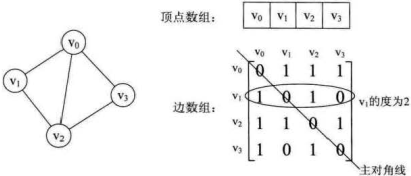• 无向图的边数组是一个对称矩阵
• 有无边：arc[i][j] 是否为 1
• 顶点的度：顶点$v_i$在邻接矩阵中第 i 行(或第 i列)的元素之和
• 邻接点：邻接矩阵中第 i 行元素值为 1 就是顶点$v_i$的邻接点。

#### 2）有向图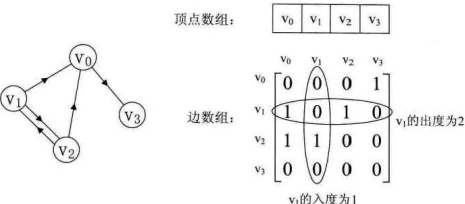• 有向图的矩阵不对称。
• 有无弧：arc[i][j] 是否为 1
• 入度：第 i 行元素之和
• 出度：第 i 列元素之和
• 邻接点：第 i 行元素值为 1 的

#### 3）网

$arc[i][j] = \begin{cases} W_ij& \text{若}(v_i,v_j)\in E \text{或} <v_i,v_j>\in E\\ 0& \text{若}i = j\\ \infty& \text{反之} \end{cases}$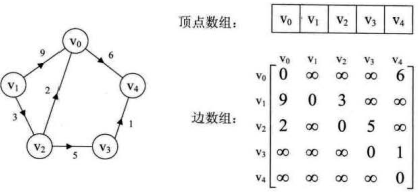### 2、邻接表：适合稀疏图

• 一维数组：存顶点，和指向第一个邻接点的指针
• 单链表：存每个顶点的所有邻接点。邻接点在顶点表中的下标，

#### 1）无向图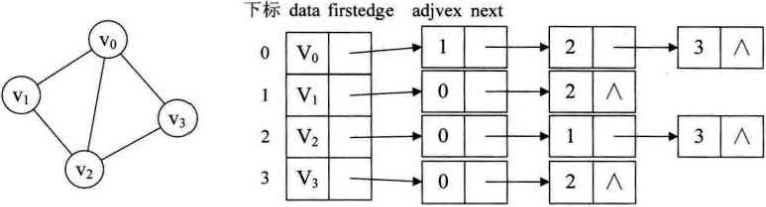• 度：顶点的边表中结点的个数
• 是否存在边：测试定点边表中是否存在结点下标
• 邻接点：顶点的边表

#### 2）有向图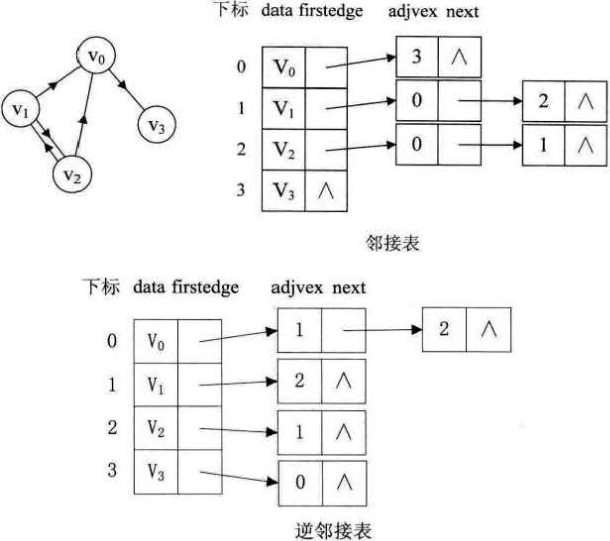#### 3）网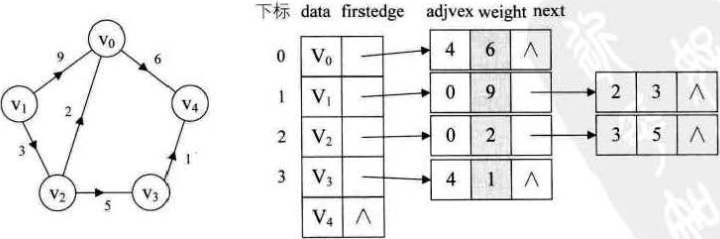### 3、十字链表：适合有向图

• 一维数组：顶点表结点
• data
• firstin：入边表头指针
• firstout：出边表头指针
• 边表结点：
• tailvex：弧起点在顶点表的下标
• weight：如果是网，存储权值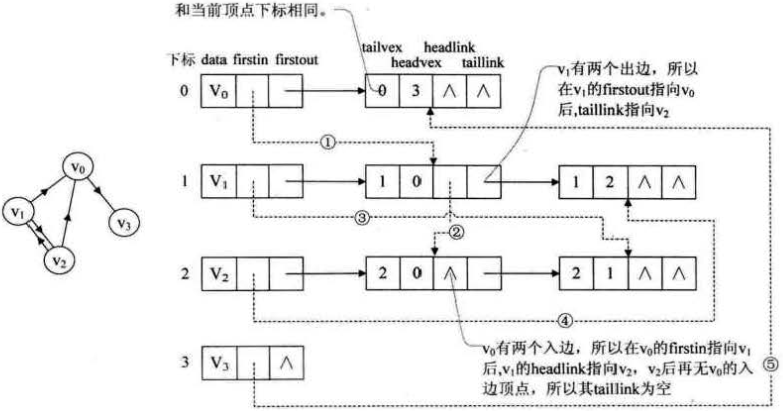### 4、邻接多重表：适合处理无向图的边

ivex 和 jvex 是与某条边依附的两个顶点在顶点表中下标。ilink 指向依附顶点 ivex 的下一条边， jlink 指向依附顶点 jvex 的下一条边。这就是邻接多重表结构。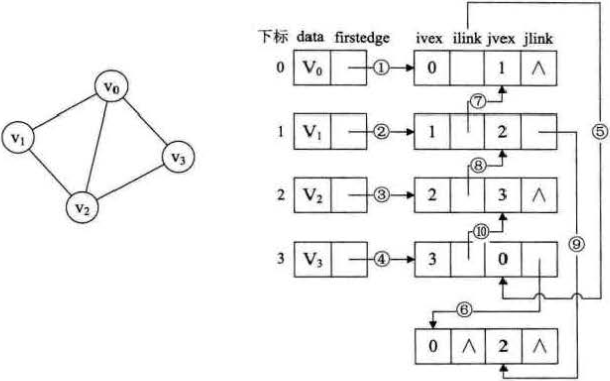### 5、边集数组：适合对边依次处理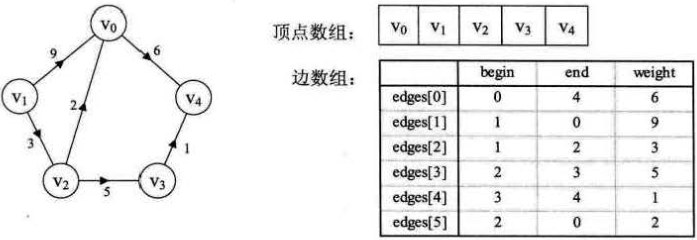## 三、图的遍历

### 1、深度优先搜索

1. 访问初始结点v，并标记结点v为已访问。
2. 查找结点v的第一个邻接结点w。
3. 若w存在，则继续执行4，否则算法结束。
4. 若w未被访问，对w进行深度优先遍历递归（即把w当做另一个v，然后进行步骤123）。
5. 查找结点v的w邻接结点的下一个邻接结点，转到步骤3。

### 2、广度优先搜索

1. 访问初始结点v并标记结点v为已访问。
2. 结点v入队列
3. 当队列非空时，继续执行，否则算法结束。
4. 出队列，取得队头结点u。
5. 查找结点u的第一个邻接结点w。
6. 若结点u的邻接结点w不存在，则转到步骤3；否则循环执行以下三个步骤：
1. 若结点w尚未被访问，则访问结点w并标记为已访问。
2. 结点w入队列
3. 查找结点u的继w邻接结点后的下一个邻接结点w，转到步骤6。

## 四、图的基本应用

### 1、最小（代价）生成树

#### 1）普里姆 ( Prim )算法

1. 将点分为两拨，已经加入最小生成树的，未加入的
2. 找到未加入中距离集合最近的点，添加该点，修改其它点到集合的距离
3. 直到所有结点都加入到最小生成树

#### 2）克鲁斯卡尔( Kruskal )算法

1. 现将所有边进行权值的从小到大排序
2. 定义一个一维数组代表连接过的边，数组的下标为边的起点，值为边的终点
3. 按照排好序的集合用边对顶点进行依次连接，连接的边则存放到一维数组中
4. 用一维数组判断是否对已经连接的边能构成回路，有回路则无效，没回路则是一条有效边
5. 重复3，4直至遍历完所有的边为止，即找到最小生成树

### 2、最短路径

#### 1）迪杰斯特拉( Dijkstra ) 算法

1. 选取初始节点作为一个集合，D(v)表示初始节点到V节点的最短路径
2. 所有能直接到达V的节点路径记为 D(v)=距离，不能直接到达的节点路径记为 D(v)=无穷
3. 选取 D(v) 最小的节点加入初始节点集合，最短路径记为D(w)=min(D(w),D(v)+j(v,w))（j(v,w)为节点V到W的距离）
4. 重复步骤3，直到所有节点都加入初始节点集合

#### 2）弗洛伊德( Floyd )算法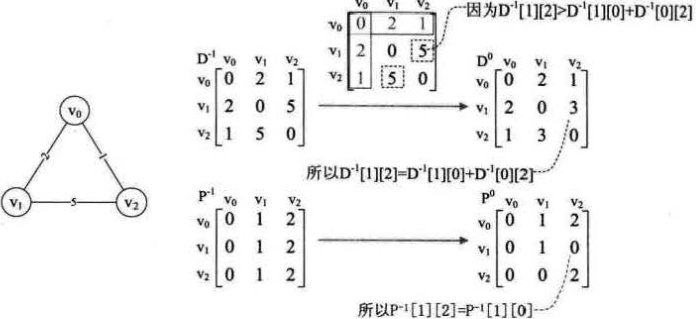1. 确定一个中间点
2. 定义两个二维数组 D[][] 和 P[][]
• D 代表顶点到顶点的最短路径权值和的矩阵，即点的邻接矩阵
• P 代表对应顶点的最小路径的前驱矩阵
3. 对于每一对顶点 v 和 w，看看是否存在一个顶点 u 使得从 v 到 u 再到 w 比己知的路径更短。如果是更新它
$D^0[v][w] = min\{ D^{-1}[v][w], D^{-1}[v]+D^{-1}[w]\}$

### 3、拓扑排序

• AOV：有向无环图
• 拓扑序列：是一个有向无环图的所有顶点的线性序列。
• 每个顶点出现且只出现一次。
• 若存在一条从顶点 A 到顶点 B 的路径，那么在序列中顶点 A 出现在顶点 B 的前面。
• 拓扑排序：对一个有向图构造拓扑序列的过程
• 从 AOV 中选择一个入度为0的顶点并输出。
• 从图中删除该顶点，井删除以此顶点为尾的弧
• 重复此步骤，直到输出全部顶点，或不存在入度为0的顶点为止。

### 4、关键路径

• AOE：有向无环网
• 路径长度：路径上各个活动所持续的时间之和
• 关键路径：从源点到汇点具有最大长度的路径
• 关键活动：在关键路径上的活动
##### 关键路径算法
1. 事件最早开始时间（etv）：顶点$v_k$最早发生的时间。
2. 事件最晚开始时间（ltv）：顶点$v_k$最晚发生的时间，超出则会延误整个工期。
3. 活动的最早开始时间（ete）：弧$a_k$最早发生时间。
4. 活动的最晚开始时间（lte）：弧$a_k$最晚发生时间。不推迟工期的最晚开工时间。

• 先要调用一次拓扑序列算法的代码来计算etv和拓扑序列表。
• 数组etv存储事件最早发生时间
• 数组ltv存储事件最迟发生时间
• 全局栈用来保存拓扑序列